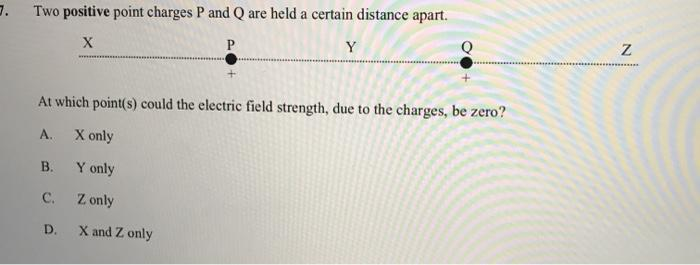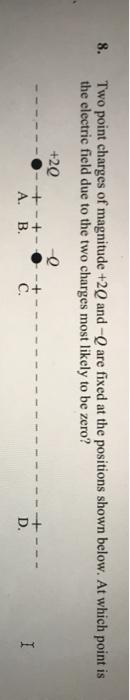# Question Solved1 Answer7. Two positive point charges P and Q are held a certain distance apart X Y Q Z At which point(s) could the electric field strength, due to the charges, be zero? A X only B. Y only C. Z only D X and Z only 8. Two point charges of magnitude +20 and are fixed at the positions shown below. At which point is the electric field due to the two charges most likely to be zero? +29 -+-+ A. B C. D. ITranscribed Image Text: 7. Two positive point charges P and Q are held a certain distance apart X Y Q Z At which point(s) could the electric field strength, due to the charges, be zero? A X only B. Y only C. Z only D X and Z only 8. Two point charges of magnitude +20 and are fixed at the positions shown below. At which point is the electric field due to the two charges most likely to be zero? +29 -+-+ A. B C. D. I
More
Transcribed Image Text: 7. Two positive point charges P and Q are held a certain distance apart X Y Q Z At which point(s) could the electric field strength, due to the charges, be zero? A X only B. Y only C. Z only D X and Z only 8. Two point charges of magnitude +20 and are fixed at the positions shown below. At which point is the electric field due to the two charges most likely to be zero? +29 -+-+ A. B C. D. I